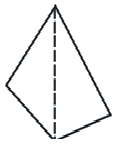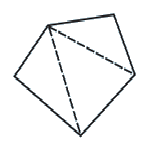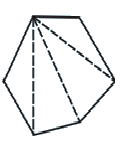# Ex.3.1 Q4 Understanding Quadrilaterals Solution - NCERT Maths Class 8

Go back to  'Ex.3.1'

## Question

Examine the table. (Each figure is divided into triangles and the sum of the angles deduced from that.)

 FIGURESSIDE 3 4 5 6 ANGLE SUM $$180$$ \begin{align} 2 \times {180^{\rm{o}}} & = (4 - 2) \times {180^{\rm{o}}}\\ &= 360^\circ \end{align} \begin{align} 3 \times {180^{\rm{o}}}\\ & = (5 - 2) \times {180^{\rm{o}}}\\ & = 540 \end{align} \begin{align} 4 \times {180^{\rm{o}}}\\ & = (6 - 2) \times {180^{\rm{o}}}\\ & = {720^{\rm{o}}} \end{align}

What can you say about the angle sum of a convex polygon with number of sides?

(a) $$7$$

(b) $$8$$

(c) $$10$$

(d) $$n$$

Video Solution
Ex 3.1 | Question 4

## Text Solution

From the table, it can be observed that the angle sum of a convex polygon of $$n$$ sides is $$\left( {n - 2} \right){\rm{ }} \times {\rm{ }}180^\circ$$

(a) When $$n = 7$$

Then Angle sum of a polygon

\begin{align} &= (n - 2) \times {180^{\rm{\circ}}} \\&= (7 - 2) \times {180^{\rm{\circ}}} \\&= 5 \times {180^{\rm{\circ}}} \\&= {900^{\rm{\circ}}}\end{align}

(b) When $$n = 8$$

Then Angle sum of a polygon

\begin{align} &= (n - 2) \times {180^{ \rm{ \circ}}} \\&= (8 - 2) \times {180^{\rm{\circ}}} \\&= 6 \times{180^{\rm{\circ}}} \\&= {1080^{\rm{\circ}}}\end{align}

(c) When $$n = 10$$

Then Angle sum of a polygon

\begin{align} &= (n - 2) \times {180^{\rm{\circ}}} \\&= (10 - 2) \times {180^{\rm{\circ}}} \\&= 8 \times {180^{\rm{\circ}}} \\&= {1440^{\rm{\circ}}}\end{align}

(d) When $$n = n$$

Then Angle sum of a polygon $$= \left( {n - {\rm{ }}2} \right) \times 180^\circ$$

Learn from the best math teachers and top your exams

• Live one on one classroom and doubt clearing
• Practice worksheets in and after class for conceptual clarity
• Personalized curriculum to keep up with school